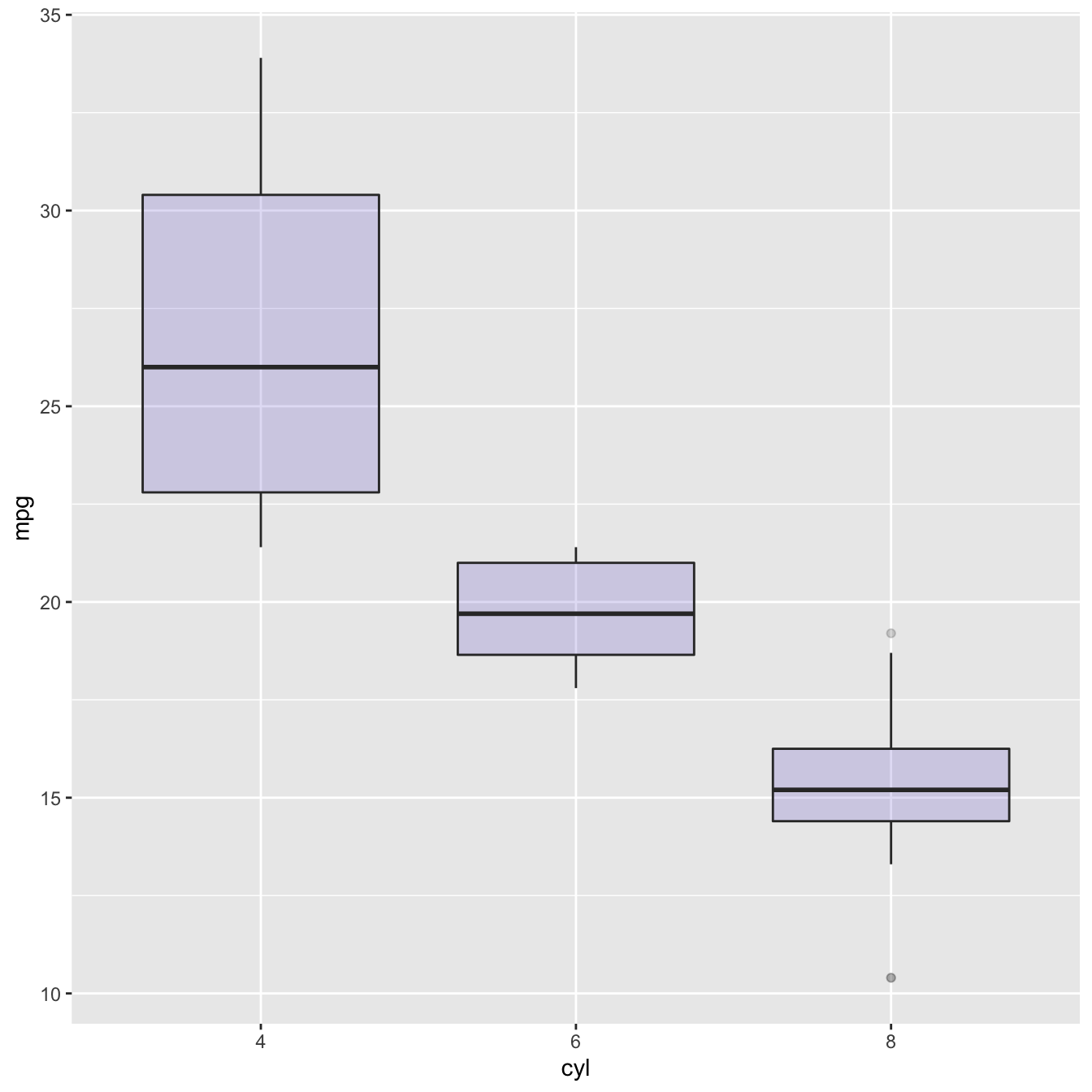# Basic ggplot2 boxplot

A boxplot summarizes the distribution of a continuous variable. It displays its median, its first and third quartiles and its outliers. Main caveat is that the underlying distribution is hidden. This page explains how to build a basic boxplot with ggplot2.

The ggplot2 library allows to make a boxplot using `geom_boxplot()`. You have to specify a quantitative variable for the Y axis, and a qualitative variable for the X axis ( a group).``````# Load ggplot2
library(ggplot2)

# The mtcars dataset is natively available

# A really basic boxplot.
ggplot(mtcars, aes(x=as.factor(cyl), y=mpg)) +
geom_boxplot(fill="slateblue", alpha=0.2) +
xlab("cyl")``````

Related chart types

## Contact

This document is a work by Yan Holtz. Any feedback is highly encouraged. You can fill an issue on Github, drop me a message on Twitter, or send an email pasting yan.holtz.data with gmail.com.